What is a number square? A number square is a grid filled with a set of numbers that can help children with a variety of mathematical concepts, including times tables and decimal numbers.

Here is an example of a number square from 1- 100: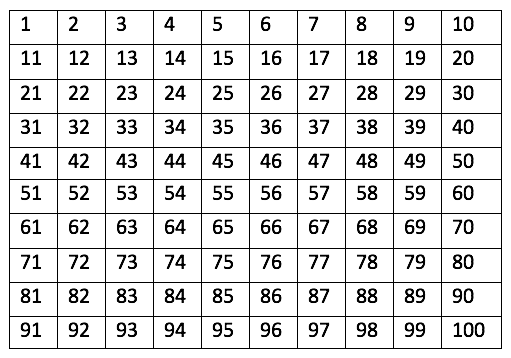Number squares are used in games like Snakes and Ladders, so it is likely that children will already be familiar with what they look like.

Number squares are not all from 1-100, for example, a number square from 1-20 may be used to help younger children count in 2s during KS1: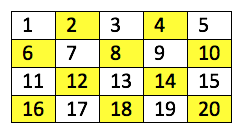Colouring in the multiples of 2 in a number grid can help children notice a pattern, like the diagonal pattern in the grid opposite. This makes the process of learning to count more memorable and enjoyable.

The numbers represented in a number grid normally follow some sort of sequence, for example they can be used to show the multiples of 5: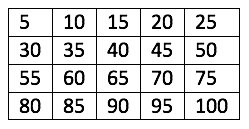## When are children taught to use number squares?

In Key Stage 1, teachers will often give children a number square from 1 to 100 and ask them to colour in the multiples of 2, 5 or 10 in different colours. Number squares could also be used in KS2 when children progress onto learning other times tables, such as 3, 4 and 6.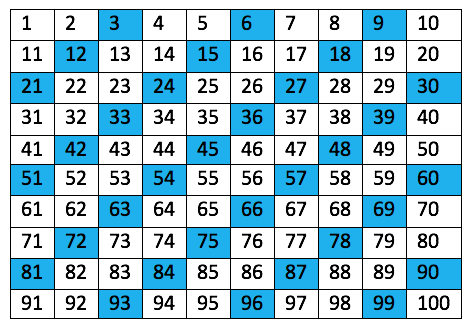For example, in this number square the multiples of 3 are coloured in blue.

Once they have completed this number square, the pattern could help children extend the number square and work out which numbers larger than 100 are a multiple of 3.

Some teachers might use number grids to demonstrate addition and subtraction. This would be helpful for adding 10 to a number because children can simply move down a row on the square to find the answer. They will learn that when adding 10 to a number, only the first digit of the number changes, e.g:

54 + 10 = 64

## Number squares can be used for percentages and decimal numbers

In Year 4 children are introduced to decimal numbers and the decimal place. Imagining a number between 1 and 0 can sometimes be a challenge for children to begin with, so teachers might use number squares to demonstrate what ‘0.1’ means.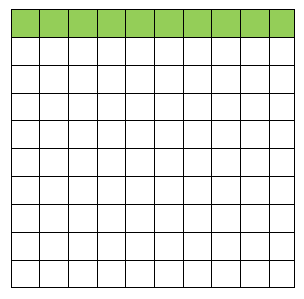To do this, teachers will give children a blank number grid which represents one whole (or 1).

They will then explain to children that each square = 0.001 (or one hundredth of a whole). Therefore, children would have to colour in 10 of these squares to represent the decimal 0.1 ( ten hundredths of a whole). This is demonstrated opposite.

Teachers might also use a number square to show children the relationship between decimals and the equivalent fractions, e.g:

0.5 = 1/2 and 0.1 = 1/10

After learning about decimals, children will be taught about percentages in Year 5. They will learn the relationship between fractions, decimals and percentages. For example:

0.5 = 50% = 1/2

0.25 = 25% = 1/4

A number square could be used to represent this relationship, like so: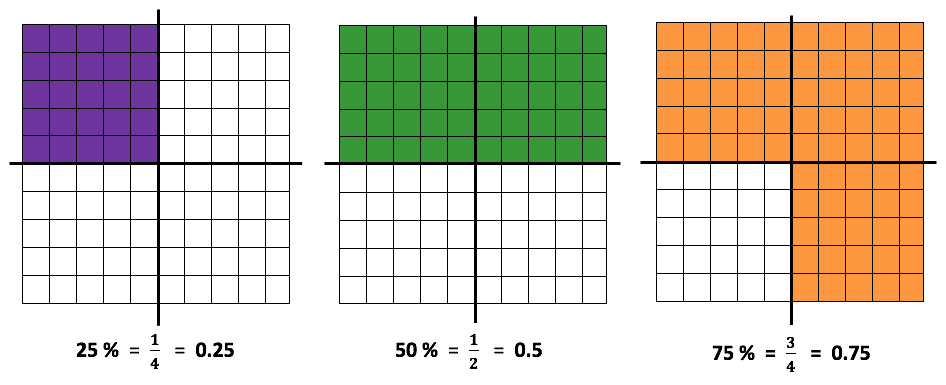## How does Learning Street help children with number squares?

Learning Street teaches introduces the concept of number squares to children and then gradually builds up their knowledge. Books and other worksheets often can’t lead to the same understanding of a subject as we can, as they cover a topic once and do not revisit it.

In fact, many tutors and tuition centres struggle to build up knowledge in such a complete way.

## Our Courses

Click through to review the courses we have available

##### Get Started

Learning Street structured courses make home study easier and more successful. There's no need to wonder which books to buy or what to do next. Everything is planned for you.

• Proven to deliver excellent results
• Used by families, private tutors and schools
• Fully planned and structured